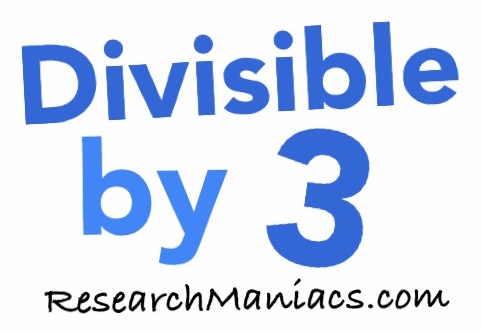Divisible by 3Congratulations! You found Research Maniacs' well-used "Divisible by 3" lookup page. When you enter a number on this lookup page, we will do three things.

1 - We will of course give you the yes/no answer to whether your number is divisible by 3 or not.

2 - We will tell you what your number divided by 3 actually is (the quotient).

3 - AND most importantly, we will show you a neat little trick for how we figure out if your number is divisible by 3, so you can use it yourself in the future without a calculator.

To get started, simply enter your number in the box below:

Is divisible by 3?

There are so many numbers to choose from, huh? If you are not sure what to enter above, give one of these a shot:

Is 46 divisible by 3?

Is 99 divisible by 3?

Is 250 divisible by 3?

Is 1765 divisible by 3?